Next: Other universal dendrites Up: Dendrites Previous: Wazewski universal dendrite

## Universal dendrites of order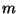Let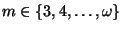. By the standard universal dendrite of orderwe mean a dendrite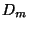such that each ramification point ofis of orderand for each arc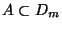the set of ramification points ofwhich belong to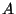is dense in. Their constructions, known again from [Wazewski 1923, Chapter K, p. 137] (see also [Charatonik 1991, (4), p. 168]; for the inverse limit construction see [Chaaratonik 1980, p. 491]), mimic that of the Wazewski universal dendrite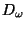, but instead of copies of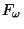we use copies of-ods at each step of the construction. See Figures A-C for the standard universal dendrites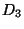,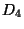and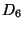.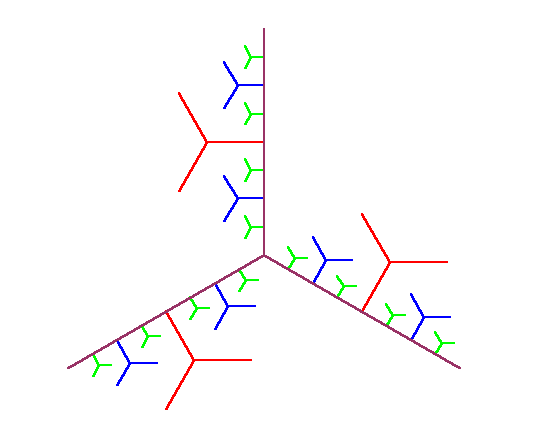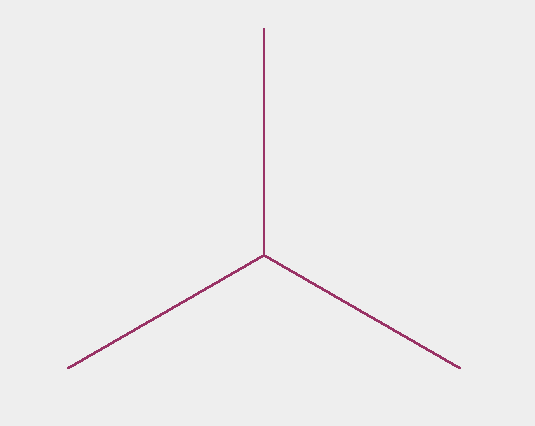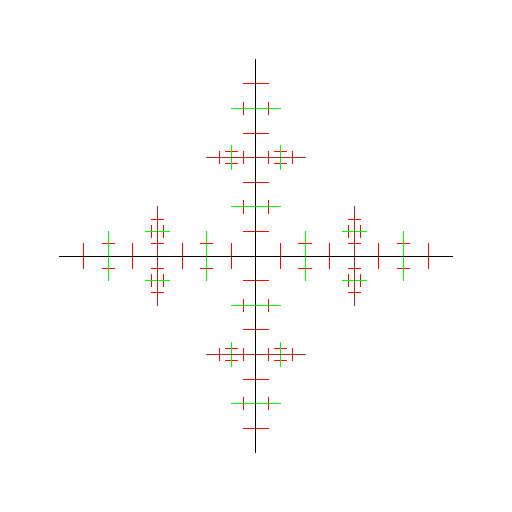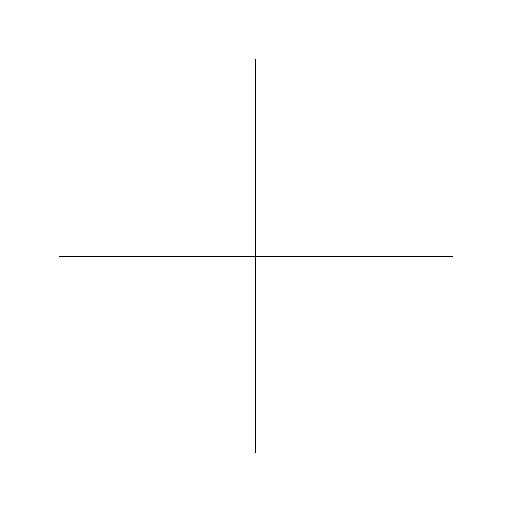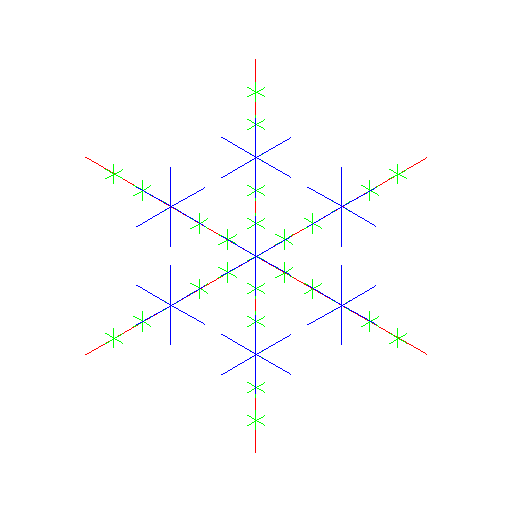The standard universal dendriteshave the following properties.

1. For eachis universal in the class of all dendrites for which the order of their ramification points is less than or equal to, see e.g. [Menger 1932, Chapter 10, § 6, p. 322].
2. If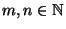with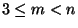, then there exists an open mapping of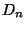onto, [Chaaratonik 1980, Theorem 2, p. 492].
3. Among all standard universal dendritesonlyandare homeomorphic with all their open images, [Chaaratonik 1980, Corollary, p. 493].
4. For eacha monotone surjection ofonto itself is a near homeomorphism if and only if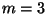, [Charatonik 1991, Corollary 5.5, p. 178].
5. Any two standard universal dendritesandof some orders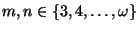are monotonely equivalent, [Charatonik 1991, Corollary 6.6, p. 180].
6. For eachthe dendriteis monotonely homogeneous [Charatonik 1991, Theorem 7.1, p. 186].

Other mapping properties of the standard universal dendritescan be found e.g. in [Chaaratonik 1980], [Charatonik 1991], [Charatonik 1995], [Charatonik et al. 1997a], [Charatonik et al. 1998], [Charatonik et al. 1994] and [Charatonik W.J. et al. 1994].

Here you can find source files of this example.

Here you can check the table of properties of individual continua.

Here you can read Notes or write to Notes ies of individual continua.Next: Other universal dendrites Up: Dendrites Previous: Wazewski universal dendrite
Janusz J. Charatonik, Pawel Krupski and Pavel Pyrih
2001-11-30